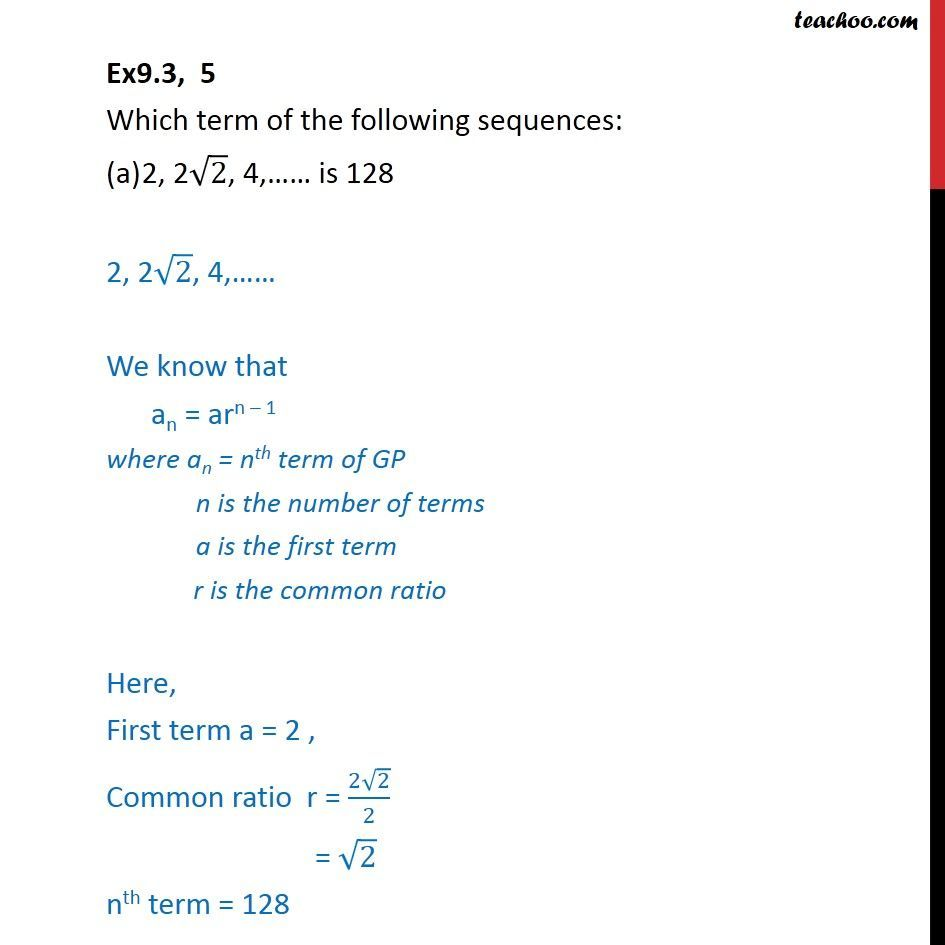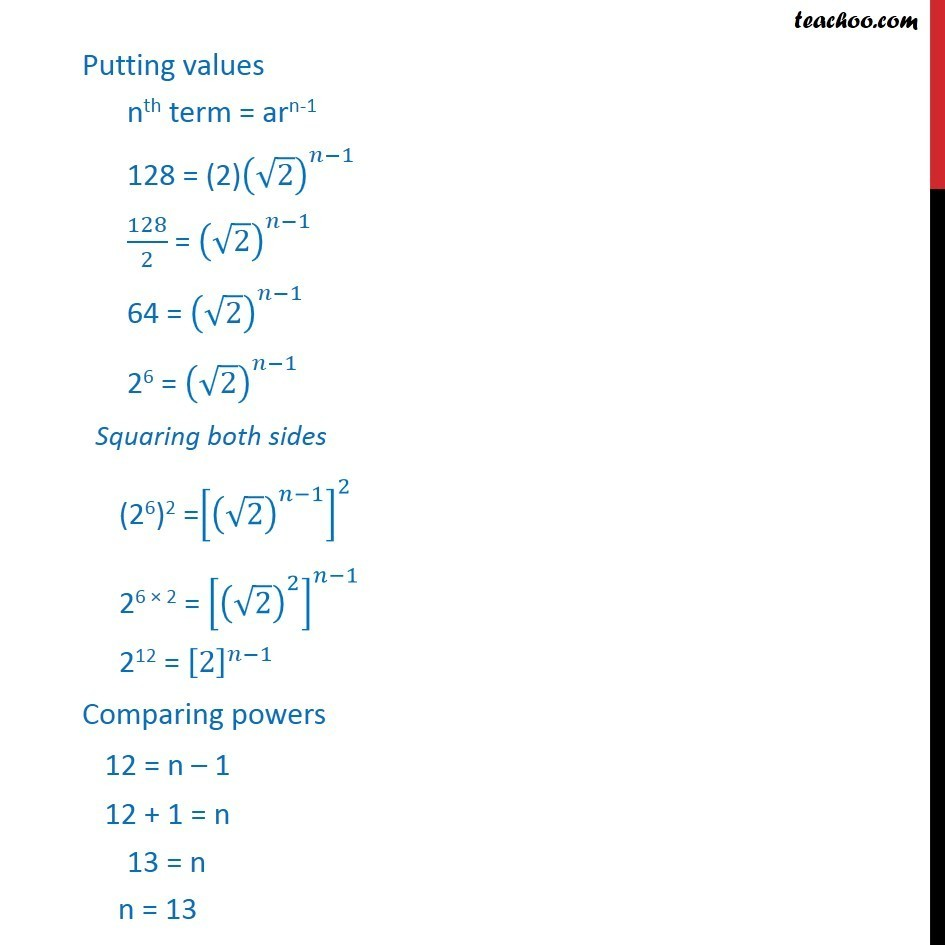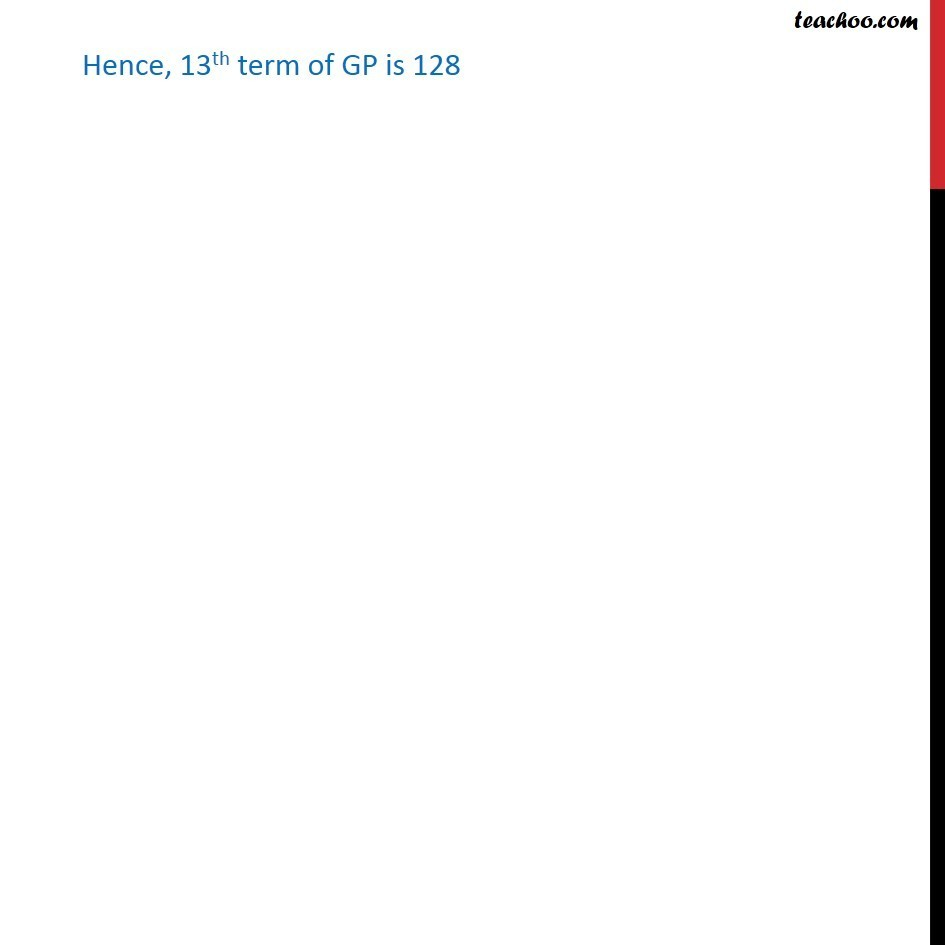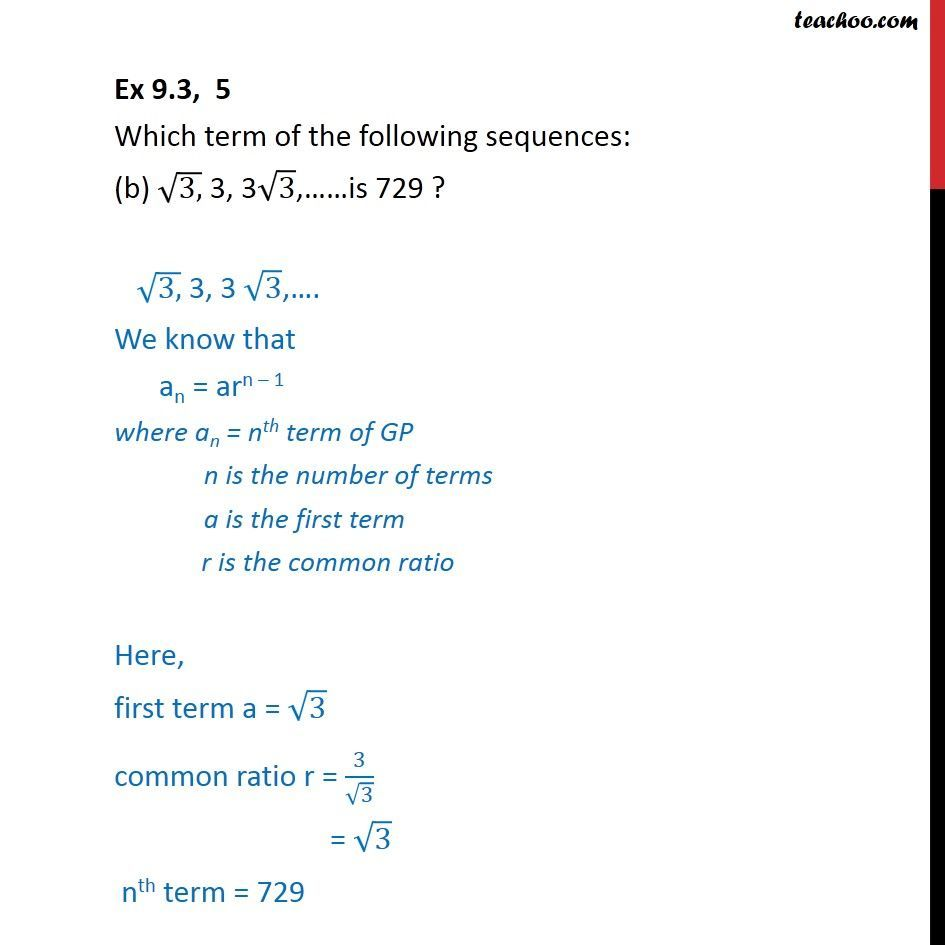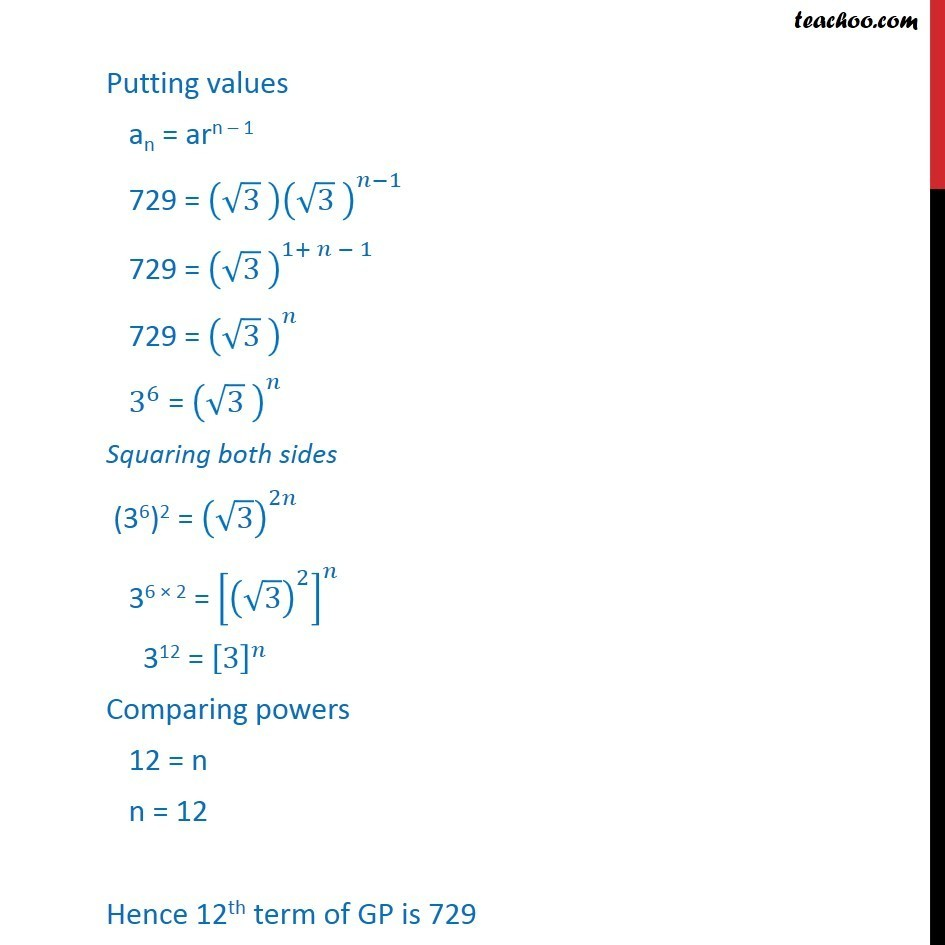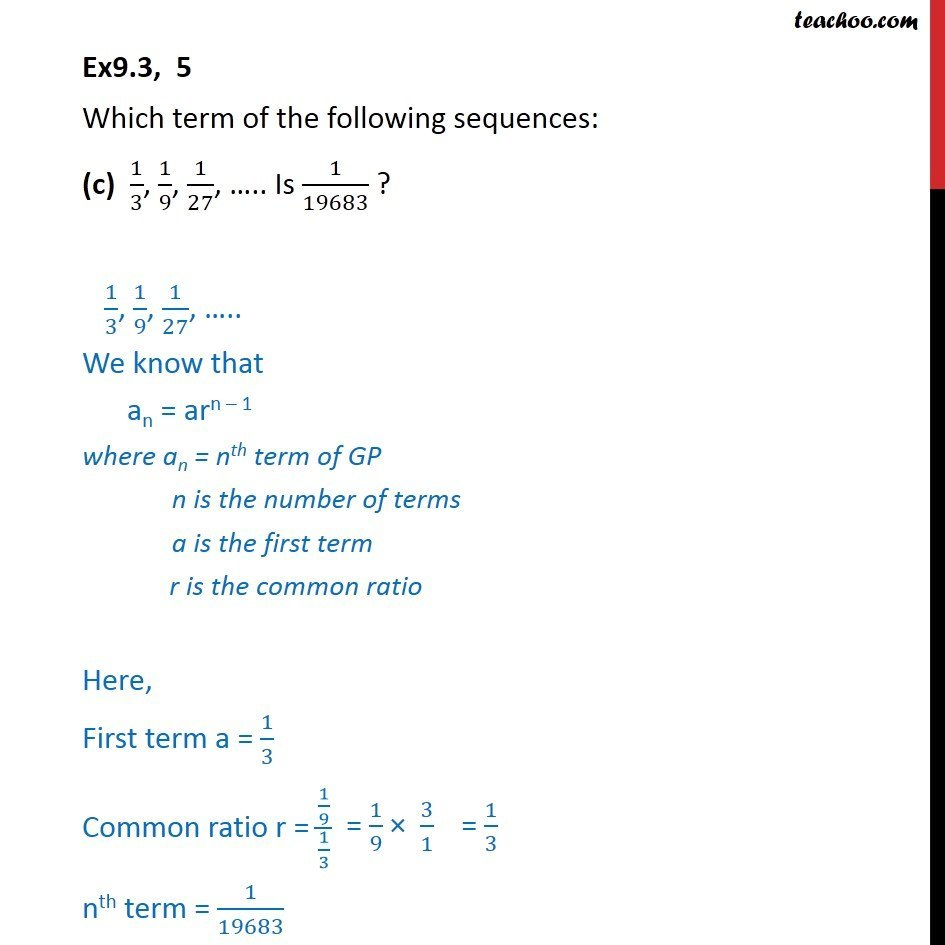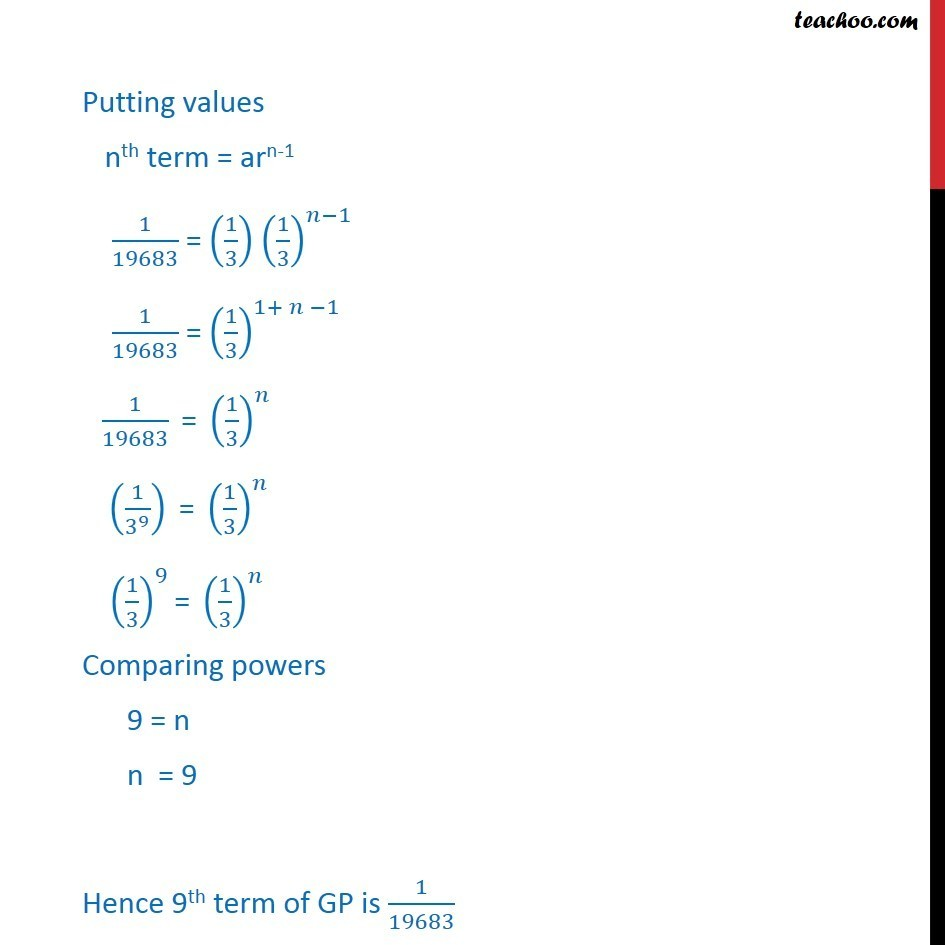Subscribe to our Youtube Channel - https://you.tube/teachoo

1. Chapter 9 Class 11 Sequences and Series
2. Serial order wise
3. Ex 9.3

Transcript

Ex9.3, 5 Which term of the following sequences: 2, 2 2, 4, is 128 2, 2 2, 4, We know that an = arn 1 where an = nth term of GP n is the number of terms a is the first term r is the common ratio Here, First term a = 2 , Common ratio r = (2 2)/2 = 2 nth term = 128 Putting values nth term = arn-1 128 = (2)( 2)^( 1) 128/2 = ( 2)^( 1) 64 = ( 2)^( 1) 26 = ( 2)^( 1) Squaring both sides (26)2 =[( 2)^( 1) ]^2 26 2 = [( 2)^2 ]^( 1) 212 = ^( 1) Comparing powers 12 = n 1 12 + 1 = n 13 = n n = 13 Hence, 13th term of GP is 128 Ex 9.3, 5 Which term of the following sequences: (b) (3,) 3, 3 3, is 729 ? (3,) 3, 3 3, . We know that an = arn 1 where an = nth term of GP n is the number of terms a is the first term r is the common ratio Here, first term a = 3 common ratio r = 3/ 3 = 3 nth term = 729 Putting values an = arn 1 729 = ( 3 ) ( 3 )^( 1) 729 = ( 3 )^(1+ 1) 729 = ( 3 )^ 3^6 = ( 3 )^ Squaring both sides (36)2 = ( 3)^2 36 2 = [( 3)^2 ]^ 312 = ^ Comparing powers 12 = n n = 12 Hence 12th term of GP is 729 Ex9.3, 5 Which term of the following sequences: (c) 1/3, 1/9, 1/27, .. Is 1/19683 ? 1/3, 1/9, 1/27, .. We know that an = arn 1 where an = nth term of GP n is the number of terms a is the first term r is the common ratio Here, First term a = 1/3 Common ratio r = (1/9)/(1/3) nth term = 1/19683 Putting values nth term = arn-1 1/19683 = (1/3) (1/3)^( 1) 1/19683 = (1/3)^(1+ 1) " " 1/19683 " = " (1/3)^ " " (1/3^9 )" = " (1/3)^ " " (1/3)^9 "= " (1/3)^ Comparing powers 9 = n n = 9 Hence 9th term of GP is 1/19683

Ex 9.3